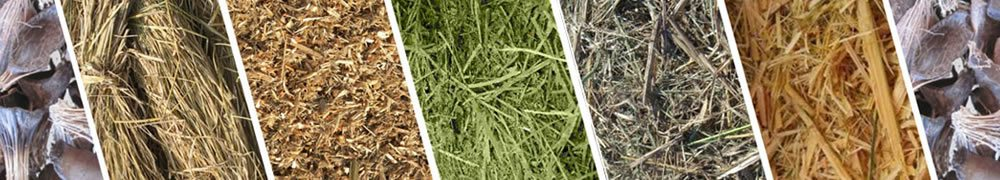﻿ Changeable Compression Ratio of Sawdust Pellet Mill - FTM China Machinery Co,.# Changeable Compression Ratio of Sawdust Pellet Mill

How to count the compression ratio of sawdust pellet mill? Compression ratios of ring die are different according to the kinds of raw materials. Taking the following materials as example. Compression ratio of poplar is 1:6. Compression ratio of deal is 1:7. For the hardwood, the compression ratio of ring die is 1:5. Compression ratios of shaving and corn straw are respectively 1:5.5 and 1:8.The compression ratios are different according to different raw materials. Harder the raw materials, smaller the compression ratio. Take the compression ratio of 1: 6 as example. What are the meanings of 1 and 6? The hole diameters of ring die are 6mm, 8mm and 10mm. Among the two numbers of 1 and 6, 1 refers to the hole diameter of 8mm.

While, 6 refers to the effective aperture of 48. Effective aperture equals to the times of hole diameter and effective hole length: 6*8=48. Then, here comes the compression ratio of 1:6=8:48. Discharging work of sawdust pellet mill is dependent on the compression ratio of ring die. Proper compression ratio of ring die can increase the output and prolong the service life.

Then, how to select the proper mold? Under the premise to use excellent ring die, we should select the proper aperture ratio and compression ratio. To ensure the strength of ring die, we should improve the aperture ratio of ring die as much as possible. Want to know more about sawdust pellet mill? Email us for more!

If you need the latest price list, please leave us a message here, thank you! We guarantee that your information will not be divulged to third parties and reply to you within 24 hours.
Tell us your needs, there will be more favorable prices!